# Excel TODAY Function

This post will guide you how to use Excel TODAY function with syntax and examples in Microsoft excel.

### Description

The Excel TODAY function returns the serial number of the current date. So you can get the current system date from the TODAY function. The serial number is the date-time code used by Excel for date and time calculations.

You can use the TODAY function to get the current date displayed on your worksheet, and regardless of when you open the workbook. And it also can be used for calculating intervals. For example, if you want to know that person’s age (he was born in 1984), you can use the following formula:

=YEAR(TODAY())-1984

This formula will use the TODAY function as an argument for the YEAR function to get the current year, and then subtracts 1984, getting his age.

The TODAY function is a build-in function in Microsoft Excel and it is categorized as a DATE and TIME Function.

The TODAY function is available in Excel 2016, Excel 2013, Excel 2010, Excel 2007, Excel 2003, Excel XP, Excel 2000, Excel 2011 for Mac.

### Syntax

The syntax of the TODAY function is as below:

`=TODAY()`

There are no any arguments for the TODAY function, but you still need to use empty parentheses ().

### Excel TODAY Function Examples

The below examples will show you how to use Excel TODAY Function to return the current system date.

1# using the TODAY function to get the current date, enter the following formula in Cell B1.

`=TODAY()`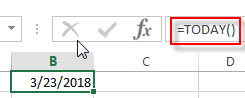2# Get the current date and then subtracts 10 days, using the following formula:

`=TODAY() -10`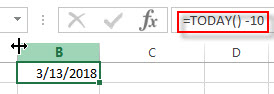3# Get the number of days between the current date and 03/14/2019, type the following formula:

`=DATEVALUE("03/14/2019")-TODAY()`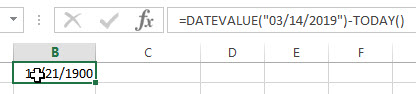4# Get the current day of the month, using the following formula:

`=DAY(TODAY())`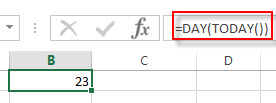5# Get the current month of the year, type the following formula in Cell B1:

`=MONTH(TODAY())`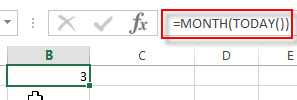You can press F9 key to force the worksheet to recalculate each time and then update the value. If the TODAY function does not update the date when you expect it to, you might need to change the settings that control when the workbook or worksheet recalculates. On the File tab, click Options, and then in the Formulas category under Calculation options, make sure that Automatic is selected.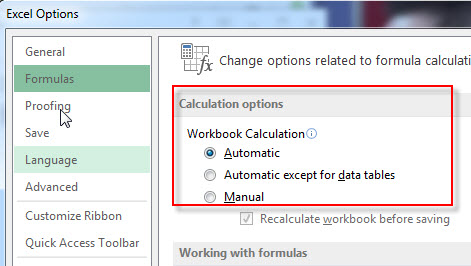### Related Functions

• Excel DAY function
The Excel DAY function returns a day of a date (from 1 to 31). The DAY function is a build-in function in Microsoft Excel and it is categorized as a DATE and TIME Function.The syntax of the DAY function is as below:= DAY (date_value)
• Excel YEAR function
The Excel YEAR function returns a four-digit year from a given date value, the year is returned as an integer ranging from 1900 to 9999. The syntax of the YEAR function is as below:=YEAR (serial_number)…
• Excel MONTH function
The Excel MONTH function returns the month of a date represented by a serial number. And the month is an integer number from 1 to 12. The syntax of the MONTH function is as below:=MONTH (serial_number)…
• Excel DATEVALUE Function
The Excel DATEVALUE function returns the serial number of date. And it can be used to convert a date represented as text format into a serial number that recognizes as a date format.The syntax of the DATEVALUE function is as below:=DATEVALUE(date_text)…

### More Excel TODAY Function Examples

• Conditional Formatting date with red Amber or Green
How to highlight the date with red if the cell dates is past now(). How to highlight the date with amber if the cell date is past now but within the next 6 months from now(). How to highlight the date with green if the cell date is more than 6 months from now…
• Highlight Rows If Dates Have Passed
Assuming that you have a worksheet that have a bunch of columns and each row has a date column. If the date in the date column goes past from now on and you want to highlight the entire row with red color….
• Count Dates in Given Year/Month/Day in Excel
You can create a formula based on the SUMPRODUCT function and the YEAR function to count dates by a give year….